# Rare Bougeoir At C C C C C C C C C C C C C C C C C C C C C C C C C C C C C C C C C C C C C C C C C C C C C C C C C C C C C C C C C C C C C C C C C C C C C C C C C C C C C C C C C C C C C C C C C C C C C C C C C C C C C C C C C C C C C C C C C C C C C C C C C C C C C C C C C C C C C C C C C C C C C C C C C C C C C C C C C C C C C C C C C C C C C C C C C C C C C C C C C C C C C C C C C C C C C C C C C C C C C C C C C C C C C C C C C C C C C C C C C C C C C C C C C C C C C C C C C C C C C C C C C C C C C C C C C C C C C C C C C C C C C C C C C C C C C C C C C C C C C C C C C C C C C C C C C C C C C C C C C C C C C C C C C C C C C C C C C C C C C C C C C C C C C C C C C C C C C C C C C C C C C C C C C C C C C C C C C C C C C C C C C C C C C C C C C C C C C C C C C C C C C C C C C C C C C C C C C C C C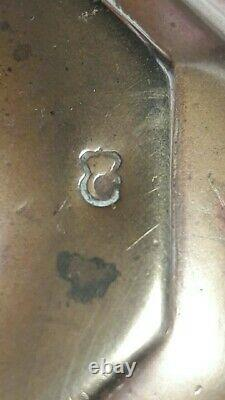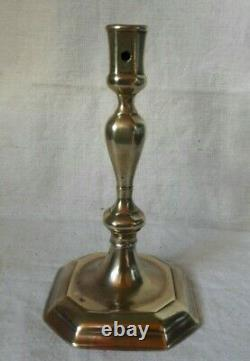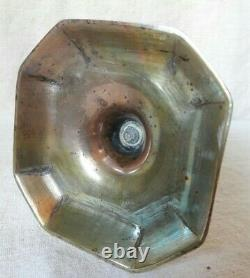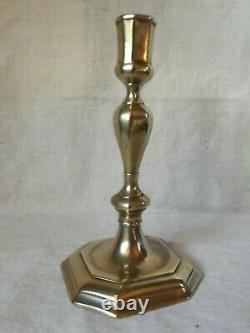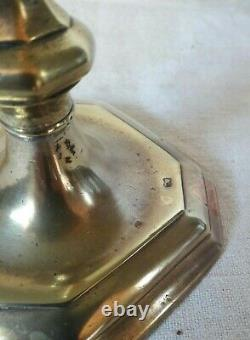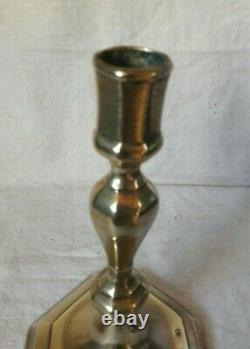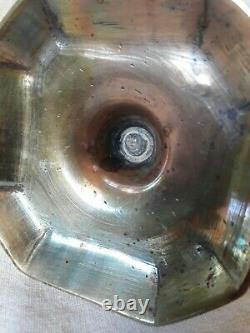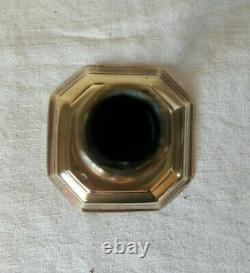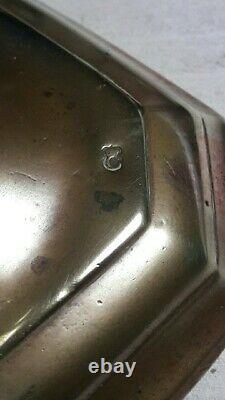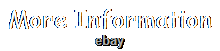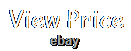Rare candlestick, molten brass candlestick in style louis xiv indicted from punch to crowned c. Condition of use, to be reported small weld reinforcement at the screw step. French work of the 18th period: (1745-1749). Size: height 18.5 cm. This item is in the category "art, antiques\ objects of xixe and before".

The seller is "fanay1972" and is located in this country: fr. This item can be shipped to the following country: European Union.

Authenticity: original
1. type: popular art object
2. Origin: france
3. subtype: candle holder
4. Material: brass Style: louis xiv, baroque